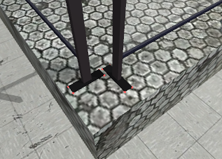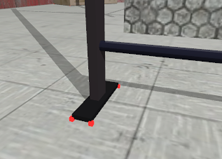Displaying contacts points between geometries.

The contacts points display option is used to reflect the complexity of the scene for the physical equations solver. The calculation time is directly dependent of the number of contact points, so it is better to minimize them (Reducing de number of contacts points, AutoDiable ...). In addition, this option is very useful to determine the geometries that are already in interpenetration at the simulation startup (ejected objects, glued ones ...).

 Couleur des points de contact DescriptionLight red color: the geometries are in contact. The physics solver computes forces/torques required for respecting the constraint at every time step.Green color: the geometries are in contact but are stabilized (Auto Disable flag). In this case the solver does not compute the forces/torques between geometries (and do not consume any CPU time). The rigid bodies will be reactivated if required (joints, contact with another rigid body that is moving etc.).Dark red color: the geometry is in contact with the 'default ground plane'. The physics solver computes forces/torques required for respecting the constraint at every time step.